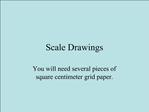# Scale Drawings - PowerPoint PPT PresentationDownload PresentationScale Drawings

Scale Drawings
Download Presentation## Scale Drawings

- - - - - - - - - - - - - - - - - - - - - - - - - - - E N D - - - - - - - - - - - - - - - - - - - - - - - - - - -
##### Presentation Transcript

1. Scale Drawings You will need several pieces of square centimeter grid paper.

2. For each shape, draw a scaled representation on your grid paper.

3. 1) A rectangular billboard is 16 ft by 12 ft. Scale: 1 cm = 4 ft

4. 1) A rectangular billboard is 16 ft by 12 ft. Scale: 1 cm = 4 ft

5. 2) A rectangular poster is 36 in by 24 in. Scale: 1 cm = 3 in

6. 2) A rectangular poster is 36 in by 24 in. Scale: 1 cm = 3 in

7. 3) A rectangular parking lot is 50 yd by 40 yd. Scale: 1 cm = 10 yd

8. 3) A rectangular parking lot is 50 yd by 40 yd. Scale: 1 cm = 10 yd

9. 4) A rectangular bulletin board is 16 ft by 10 ft. Scale: 1 cm = 2 ft

10. 4) A rectangular bulletin board is 16 ft by 10 ft. Scale: 1 cm = 2 ft

11. 5) A rectangular playground is 80 ft by 60 ft. Determine your own scale: 1 cm = ____ ft

12. 5) A rectangular playground is 80 ft by 60 ft. Determine your own scale: 1 cm = 10 ft

13. 6) A rectangular hallway is 8 ft by 20 ft. Determine your own scale: 1 cm = _____ ft

14. 6) A rectangular hallway is 8 ft by 20 ft. Determine your own scale: 1 cm = 4 ft

15. 7) A rectangular room is 12 ft by 24 ft. Determine your own scale: 1 cm = ____ ft

16. 7) A rectangular room is 12 ft by 24 ft. Determine your own scale: 1 cm = 4 ft

17. 8) A rectangular swimming pool is 50 meters by 30 meters. Determine your own scale: 1 cm = ____ m

18. 8) A rectangular swimming pool is 50 meters by 30 meters. Determine your own scale: 1 cm = 10 m

21. Tell whether the scale reduces, enlarges, or preserves the size of an actual object.

22. Tell whether the scale reduces, enlarges, or preserves the size of an actual object.

23. Tell whether the scale reduces, enlarges, or preserves the size of an actual object.

30. The End.